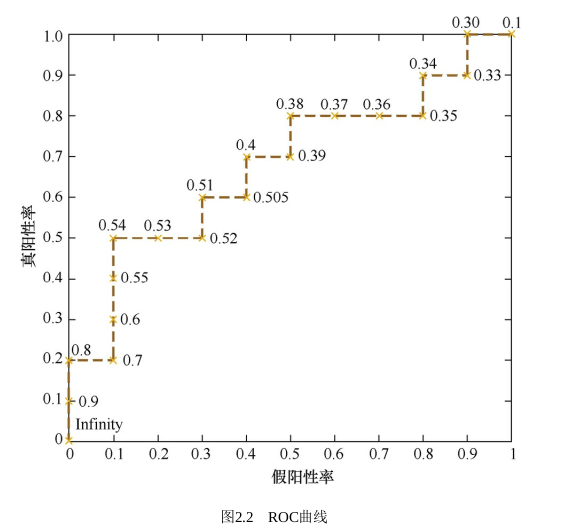始

“You are writing a book because you are not entirely satisfied with the available texts.” – George Casella

When&Where&Who&Why

The ROC curve was first used during World War II for the analysis of radar signals before it was employed in signal detection theory. Following the attack on Pearl Harbor in 1941, the United States army began new research to increase the prediction of correctly detected Japanese aircraft from their radar signals. For these purposes they measured the ability of a radar receiver operator to make these important distinctions, which was called the Receiver Operating Characteristic. --Wikipedia

ROC曲线最早是运用在军事上的,后来逐渐运用到医学领域,并于20世纪80年代后期被引入机器学习领域。相传在第二次 世界大战期间,雷达兵的任务之一就是死死地盯住雷达显示器,观察是否有敌机来袭。理论上讲,只要有敌机来袭,雷达屏幕上 就会出现相应的信号。但是实际上,如果飞鸟出现在雷达扫描区域时,雷达屏幕上有时也会出现信号。这种情况令雷达兵烦恼不

What

A receiver operating characteristic curve, or ROC curve, is a graphical plot that illustrates the diagnostic ability of a binary classifier system as its discrimination threshold is varied. --Wikipedia

Wiki上明确地给出我前面说的“某样东西”是一个"binary classifier sysytem"， 即一个二分类系统(再次强调，请不要局限在ML里面的二分类…一个分辨灯泡好坏的仪器同样是一个二分类系统，这在质量管理中的例子很多).

TP, FP, TN, FN: True Positive, False Positive, True Negative, False Negative

TPR: True Postive Rate, Recall, Sensitivity, $$TPR = \frac{TP}{TP+FN}$$

FPR: False Positive Rate, Fall-out, $FPR=\frac{FP}{FP+TN}$

ROC定义横轴为FPR，纵轴为TPR。进而问题转化成如何从Threshold $T$得到对应点的横纵座标，即$(FPR_T， TPR_T)$.从某个集合$S_T$中取不同的阈值$T$，就得到一系列的横纵座标，也就是ROC曲线上的一系列点${(FPR_T, TPR_T) | T \in S_T}$, 也就得到了ROC曲线。AUC

AUC的概率解释在Wikipedia中有详细的推导.
\begin{equation}\begin{aligned} &T P R(T): T \rightarrow y(x)\ &F P R(T): T \rightarrow x\ &\mathrm{TPR}(T)=\int_{T}^{\infty} f_{1}(x) d x\ &\operatorname{FPR}(T)=\int_{T}^{\infty} f_{0}(x) d x\ &AUC=\int_{x=0}^{1} \operatorname{TPR}\left(\operatorname{FPR}^{-1}(x)\right) d x=\int_{\infty}^{-\infty} \operatorname{TPR}(T) \operatorname{FPR}^{\prime}(T) d T=\int_{-\infty}^{\infty} \int_{-\infty}^{\infty} I\left(T^{\prime}>T\right) f_{1}\left(T^{\prime}\right) f_{0}(T) d T^{\prime} d T=P\left(X_{1}>X_{0}\right) \end{aligned}\end{equation}

$$A U C(f)=\frac{\sum_{t_{0} \in \mathcal{D}^{0}} \sum_{t_{1} \in \mathcal{D}^{1}} \mathbf{1}\left[f\left(t_{0}\right)<f\left(t_{1}\right)\right]}{\left|\mathcal{D}^{0}\right| \cdot\left|\mathcal{D}^{1}\right|}$$

When using normalized units, the area under the curve (often referred to
as simply the AUC) is equal to the probability that a classifier will
rank a randomly chosen positive instance higher than a randomly chosen
negative one (assuming ‘positive’ ranks higher than ‘negative’)